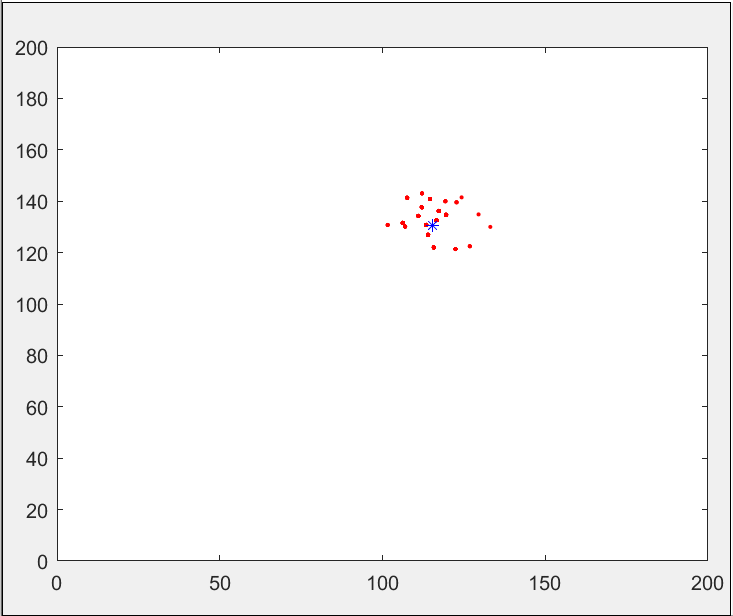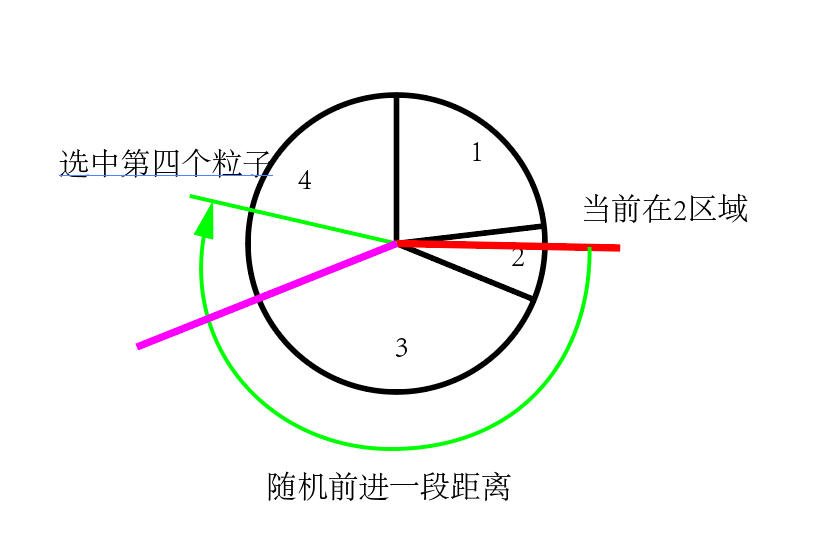## 粒子滤波器的Matlab实现_matlab 粒子滤波器_木独的博客-程序员秘密# 原理介绍

## 算法流程

1. 根据上一次的粒子分布和控制命令更新粒子位置
2. 对更新后的粒子计算在该粒子的情况下，获得传感器观测的概率作为权重
3. 根据权重进行重采样

## 一、根据上一次分布和控制指令更新粒子位置

%控制机器人前进
function pos = move(robot_pos,set_w,set_v,control_noise)
% 先进行旋转,加上噪声，并归一化到0~pi*2
pos(3) = mod(robot_pos(3) + set_w + control_noise*rand(),2*pi);
% 前进距离+噪声
V = set_v + control_noise*rand();
% 坐标变换，因为前进距离是车体坐标系而要求的是世界坐标系下的
pos(1) = mod(robot_pos(1) - V*sin(pos(3)),200);
pos(2) = mod(robot_pos(2) + V*cos(pos(3)),200);
end

## 二、对更新后的粒子计算在该粒子的情况下，获得传感器观测的概率作为权重

% 获取机器人在当前位置观测到对应数据的概率
function weight = get_probability(P,Z,landMark,sensor_noise)
particle_Z = getDistance(P,landMark,sensor_noise);%根据地图获取理论上最有可能的观测结果
distance = particle_Z - Z;%计算和真实值之间的差值
noise = zeros([1,12]) + sensor_noise*sensor_noise;%根据设定观测噪声，构建协方差
sigma = diag(noise);
%带入多维高斯分布的公式中，这里去掉了归一化常数项
weight = exp(-1/2 * (distance)' / (sigma) * (distance));
end

## 三、根据权重进行重采样% 粒子滤波：根据权重进行重采样
sum_weight = sum(weight);
weight = weight./sum_weight;%归一化权重
max_weight = max(weight);
index = ceil(rand()*particle_num);%开始时随机选取一个位置
for i = 1:particle_num %随机采样的过程
beta = rand() * 2 * max_weight;%随机生成前进距离，避免太远处或太近，设置为最大值的两倍
while beta > weight(index+1)%重采样过程
beta = beta - weight(index+1);
index = mod(index + 1,particle_num-1);
end
temp_particle(:,i) = particle(:,index+1);
end
particle = temp_particle;

# Matlab代码实现

%% begin
clc;
clear;
%% set param
map_length = 200;%场地范围为200m
% 路标
landMarkNum = 12;%设定12个路标
%随机生成路标作为地图
landMark = [rand()*map_length rand()*map_length rand()*map_length rand()*map_length rand()*map_length rand()*map_length rand()*map_length rand()*map_length rand()*map_length rand()*map_length rand()*map_length rand()*map_length
rand()*map_length rand()*map_length rand()*map_length rand()*map_length rand()*map_length rand()*map_length rand()*map_length rand()*map_length rand()*map_length rand()*map_length rand()*map_length rand()*map_length];
control_noise = 5; %控制机器人运动的噪声
sensor_noise = 10; %传感器对地图的测量噪声
robot_v = 20; %机器人在20m内随机运动
robot_w = 0;%0.1*pi;
T = 20;%进行T次迭代
particle_num = 1000;%使用1000个粒子
particle = zeros([3,particle_num]);%粒子的位置
temp_particle = zeros([3,particle_num]);%更新粒子时的临时变量
weight = zeros([1,particle_num]);%粒子的权重
%% 粒子滤波步骤
% 随机生成初始机器人的位置
robot_pos = [rand()*map_length,rand()*map_length,rand()*2*pi]';
% 随机生成初始粒子分布的位置
for i = 1:particle_num
particle(:,i) = [rand()*map_length,rand()*map_length,rand()*2*pi]';
end
%% plot
plot(robot_pos(1),robot_pos(2),'b*');
xlim([0 200])
ylim([0 200])
hold on;
for i=1:landMarkNum
plot(landMark(1,i),landMark(2,i),'go');
hold on;
end
for i=1:particle_num
plot(particle(1,i),particle(2,i),'y.');
hold on;
end
for t = 1:T
% 假设机器人一直在随机运动
set_v = rand()*robot_v;
set_w = rand()*robot_w;
% 将命令给实际的机器人，但是会有一定的误差
robot_pos = move(robot_pos,set_w,set_v,control_noise/10);
% 在当前位置测量,测量同样会有一定误差
Z = getDistance(robot_pos,landMark,sensor_noise);
% 粒子滤波：根据上一次的分布和当前指令得到预测分布，并计算权重
for i = 1:particle_num
particle(:,i) = move(particle(:,i),set_w,set_v,control_noise);
weight(i) = get_probability(particle(:,i),Z,landMark,sensor_noise);
end
% 粒子滤波：根据权重进行重采样
sum_weight = sum(weight);
weight = weight./sum_weight;%归一化权重
max_weight = max(weight);
index = ceil(rand()*particle_num);%开始时随机选取一个位置
for i = 1:particle_num %随机采样的过程
beta = rand() * 2 * max_weight;%随机生成前进距离，避免太远处或太近，设置为最大值的两倍内
while beta > weight(index+1)%重采样过程
beta = beta - weight(index+1);
index = mod(index + 1,particle_num-1);
end
temp_particle(:,i) = particle(:,index+1);
end
particle = temp_particle;
%% update plot
clf;
for i=1:particle_num
plot(particle(1,i),particle(2,i),'r.');
hold on;
end
plot(robot_pos(1),robot_pos(2),'b*');
xlim([0 200])
ylim([0 200])
hold on;
pause(0.1);
end

%% function define
% 获取机器人在当前位置观测到对应数据的概率
function weight = get_probability(P,Z,landMark,sensor_noise)
particle_Z = getDistance(P,landMark,sensor_noise);%根据地图获取理论上最有可能的观测结果
distance = particle_Z - Z;%计算和真实值之间的差值
noise = zeros([1,12]) + sensor_noise*sensor_noise;%根据设定观测噪声，构建协方差
sigma = diag(noise);
%带入多维高斯分布的公式中，这里去掉了归一化常数项
weight = exp(-1/2 * (distance)' / (sigma) * (distance));
end
%计算当前机器人位置观测到地图中的地标位置的距离
function distance = getDistance(position,landMark,sensor_noise)
dis = [0,0,0,0,0,0,0,0,0,0,0,0]';
for j=1:length(landMark) %遍历所有地标
% 计算和当前机器人的距离
l = sqrt((position(1) - landMark(1,j))^2 + (position(2) - landMark(2,j))^2);
dis(j) = l + sensor_noise*rand();% 加上随机生成的噪声项
end
% 返回各距离数据
distance = dis;
end

%控制机器人前进
function pos = move(robot_pos,set_w,set_v,control_noise)
% 先进行旋转,加上噪声，并归一化到0~pi*2
pos(3) = mod(robot_pos(3) + set_w + control_noise*rand(),2*pi);
% 前进距离+噪声
V = set_v + control_noise*rand();
% 坐标变换，因为前进距离是车体坐标系而要求的是世界坐标系下的
pos(1) = mod(robot_pos(1) - V*sin(pos(3)),200);
pos(2) = mod(robot_pos(2) + V*cos(pos(3)),200);
end


### [IE编程] IE 版本号大全_云深互联的博客-程序员秘密

IE 所有的历史版本的版本号都可以在这里查到： http://support.microsoft.com/kb/164539  最近的 IE8 RC1 的版本号(8.0.6001.18372) 还没有加进去。 Version          Product   ------------------------------------------------------------

### 去IOE（IBM服务器,Oracle，EMC存储），Oracle迁移postgreSQL部分问题解决方案_去dell ibm oracle_dzg红茶的博客-程序员秘密

Oracle迁移postgreSQL部分问题解决方案 “去IOE化"理念最早是由阿里巴巴提出。具体就是要把公司里IBM服务器、Oracle数据库、EMC存储都替换掉， 更换为开源或国产化的选项，postgreSQL 对Oracle兼容性比较好。1.当前时间 oracle的sysdate处理方法：未开启事务时用now()替换，一般项目也是没有事务的。在事务中用到sysdate时，建议用clock_timestamp()替换。例子select sysdate from dual 等价于

### awstats分析nginx日志文件_chlinwei的博客-程序员秘密

awstats分析nginx日志文件,将生成的结果(为txt文件)保存在/var/www/awstats目录下,通过apche来显示生成的结果。nginx的域名为:www.a.com:80 LogFile="/usr/local/mybin/nginx/logs/access.log"  #nginx的日志文件路径DirData="/var/www/awstats/"

### drop index if exists mysql_mysql判断索引是否存在时删除索引的方法(mysql drop index if exists PROCEDURE)..._鹊华清扬的博客-程序员秘密

mysql判断索引是否存在时删除索引的方法(mysql drop index if exists PROCEDURE)mysql的drop index语句不支持if exists条件，在sql中先删除索引，再创建索引，如果对于新建的数据库，库中没有该索引，就会报错，导致后面的sql不再执行。因此需要使用存储过程来判断索引是否存在，如果存在则删除。sql代码如下：Sql代码DROP PROCEDUR...

### qcc514x-qcc304x调试笔记-charger_leezs0614的博客-程序员秘密

adk\src\domains\power\charger_monitor\charger_monitor_config.h/*! The time to debounce charger state changes (ms). The charger hardware will have a more limited range. */#define appConfigChargerStateChangeDebounce() (128)/*! Trickle-cha

### pygame 安装_baimi1963的博客-程序员秘密

1.https://bitbucket.org/pygame/pygame/downloads/ 确认自己需要的版本2. win 用管理员账号运行 cmd py -m pip install -U pygame --userpygame-1.9.2-cp35-cp35m-macosx_10_9_intel.whl (自动下的比我看到的还新）...